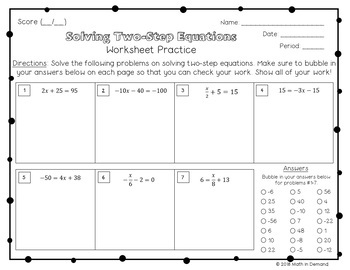### 7th Grade Math Practice Worksheets

This includes a whole years worth of practice worksheets for 7th grade math. These worksheets are self-assessing because they include a bubble-in area for the answer key. There are over 60 worksheets! I have broken up my worksheets into 5 units. These 5 units are:Unit 1 - Number Sense

Unit 2 - Ratios & Proportional Relationships

Unit 3 - Expressions & Equations

Unit 4 - Geometry

Unit 5 - Statistics & ProbabilityA breakdown of each unit is below. There will be a worksheet for every concept below:

Unit 1 - Number Sense

-Absolute Value

-Numbers on the Number Line

-Adding & Subtracting Integers on the Number Line

-Adding & Subtracting Fractions on the Number Line

-Adding & Subtracting Integers using Visual Representation

-Adding & Subtracting Integers using Rules

-Multiplying Integers

-Dividing Integers

-Least Common Multiple & Greatest Common Factor

-Multiplying Fractions

-Dividing Fractions

-Multiplying Decimals

-Dividing Decimals

Unit 2 - Ratios & Proportional Relationships

-Intro to Ratios

-Intro to Rates

-Unit Rates Word Problems

-Writing Proportions

-Solving Proportions

-Proportional Relationships Equation

-Proportional Relationship in a Table and Graph

-Unit Rate on a Graph

-Converting between Decimals, Fractions, and Percents

-Markdowns/Discounts

-Markups

-Sales Tax

-Tip

-Commission

-Percent Increase/Decrease

-Simple Interest

Unit 3 - Expressions & Equations

-Combining Like Terms

-Distributive Property

-Simplifying Expressions

-Solving One-Step Equations

-Solving Two-Step Equations with Modeling

-Solving Two-Step Equations

-Solving and Graphing One-Step Inequalities

-Solving and Graphing Two-Step Inequalities

Unit 4 - Geometry

-Constructing Triangles

-Intro to Angle Relationships

-Evaluating Angles

-Identifying Angle Relationships

-Solving with Angle Relationships

-Rectangles: Area and Perimeter

-Triangles: Area and Perimeter

-Circles: Area and Circumference

-Similar Figures

-Scale Drawings

-Volume

-Surface Area using Nets

Unit 5 - Statistics & Probability

-Random Sampling

-Draw Inferences

-Numerical Data Distributions

-Mean, Median, Mode, and Range

-Mean Absolute Deviation

-Line Plots

-Stem and Leaf Plot

-Box and Whisker Plot

-Probability of an Event

-Probability of a Repeated Event

-Probability of Independent and Dependent Events

-Likelihood